07/28 20:35

# 1. 生成式AI如何在智能家居中落地？

7月12日，思必驰推出了自研对话式大模型DFM-2（Dialogue Foundation Model），中文直译为“通用对话基础模型”。未来将围绕“云+芯”战略，以对话式AI为核心，将DFM-2大模型技术与综合全链路技术进行结合，不断提升AI软硬件产品的标准化能力和DUI平台的规模化定制能力，快速满足智能汽车、智能家居、消费电子，以及金融、轨交、政务等数字政企行业场景客户的复杂个性化需求，打造行业语言大模型，赋能产业升级。

## 3. AI大模型在医疗领域起飞

ChatGPT等大型语言模型在语言理解、生成、知识推理等方面正展现出令人惊艳的能力。近段时间，各企业开始探索大模型在不同行业中的应用落地，并针对不同领域推出相对应的行业大模型，包括在医疗领域。

## 4. GPT-4里套娃LLaMA 2！OpenAI创始成员周末爆改「羊驼宝宝」，GitHub一日千星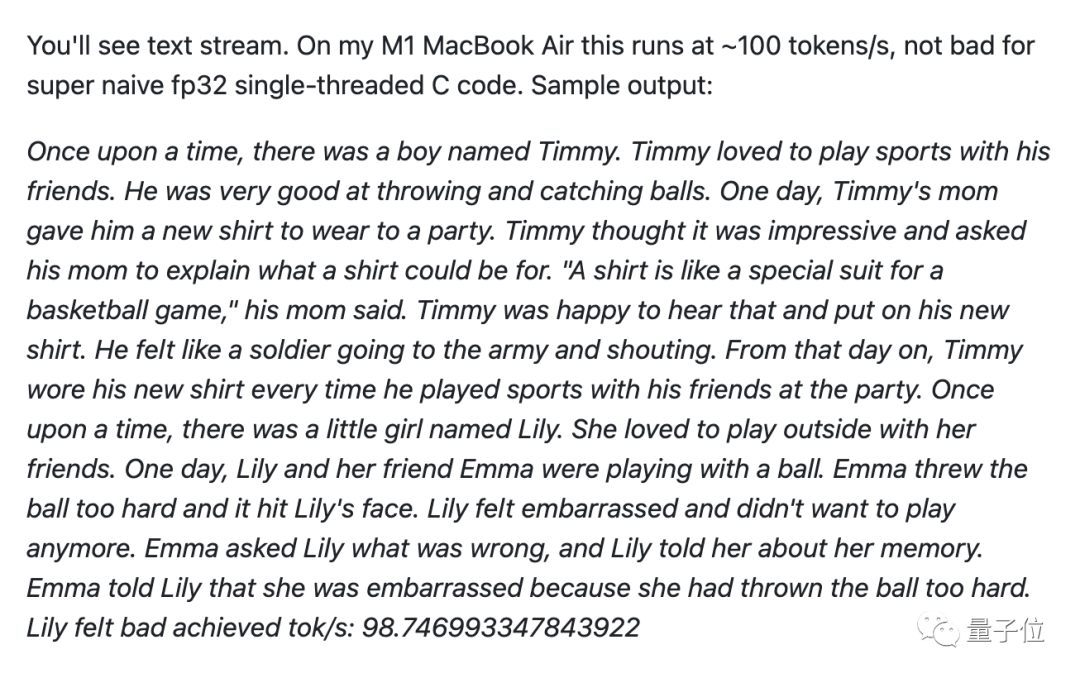llama.cpp的作者Georgi Gerganov搞出了直接在浏览器里运行的版本。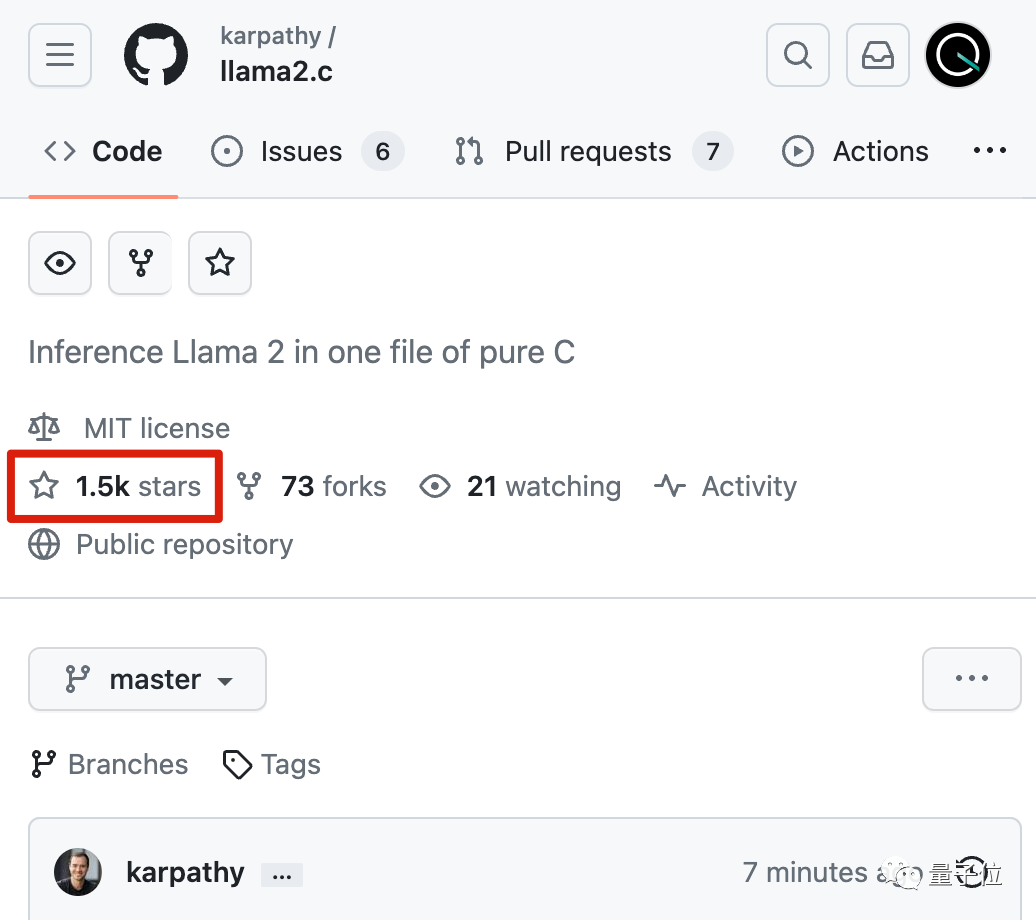2023新视野数学奖得主Ronen Eldan、2023斯隆研究奖得主李远志联手，验证了**1000万参数以下的小模型，在垂直数据上训练也可以学会正确的语法、生成流畅的故事、甚至获得推理能力。**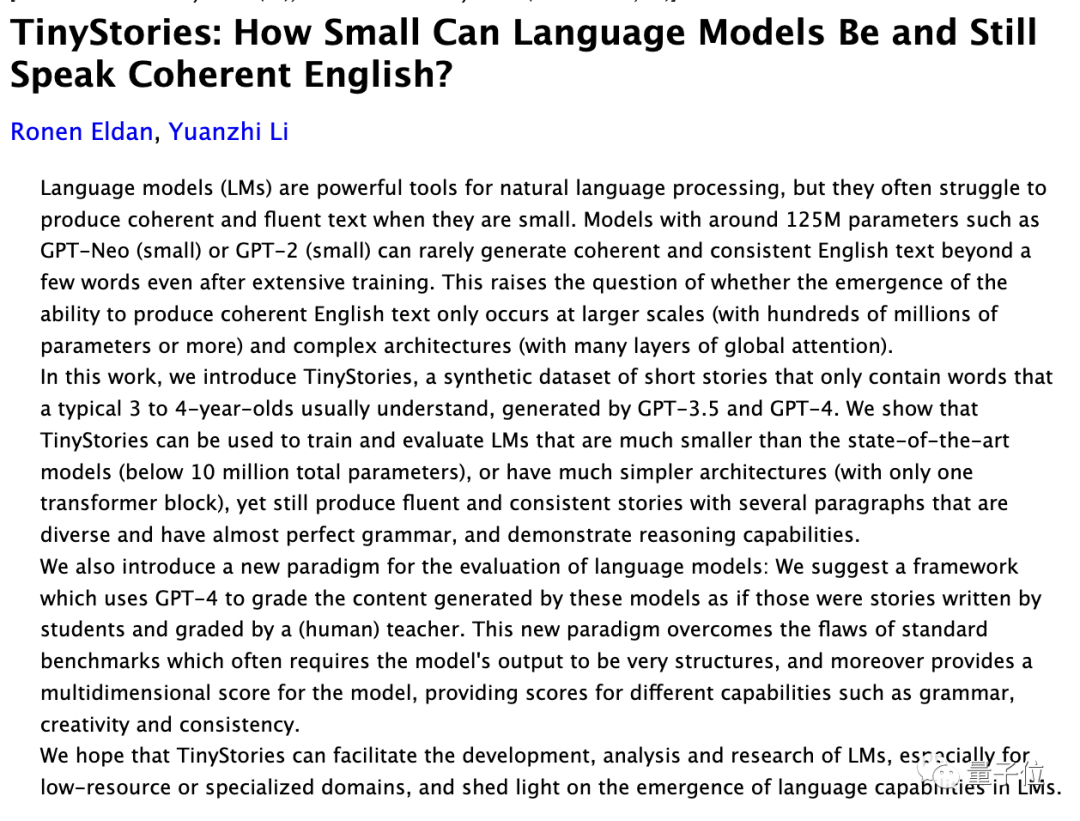Karpathy很久不写C语言已经生疏了，但是在GPT-4的帮助下，还是只用一个周末就完成了全部工作。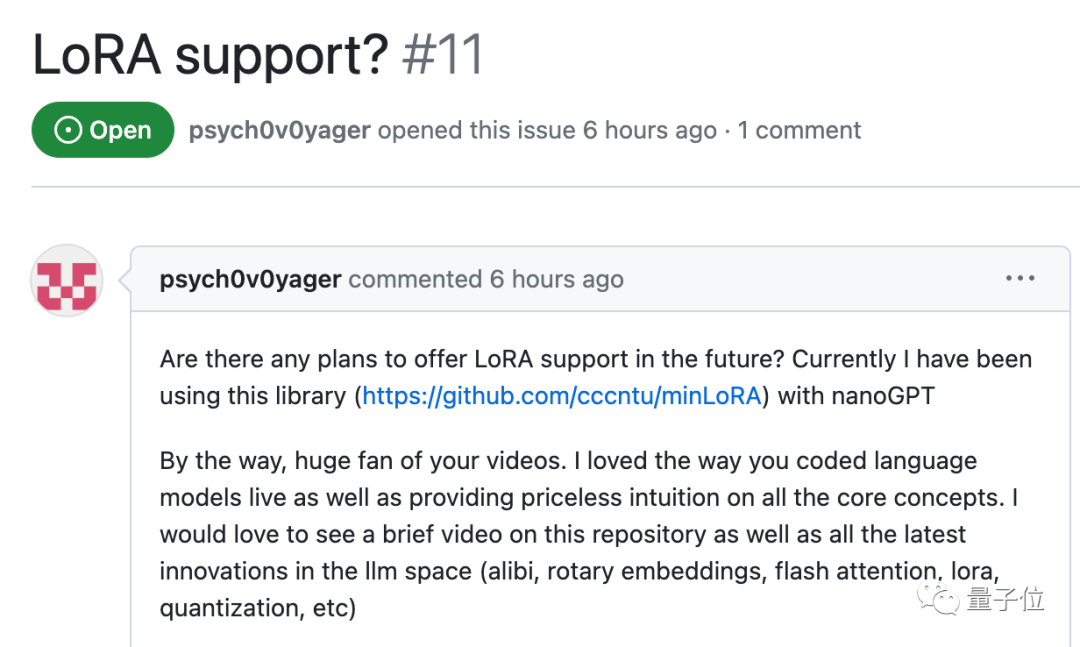Baby LLaMA 2一路火到Hacker News社区，也引发了更多的讨论。

70亿参数也许触手可及。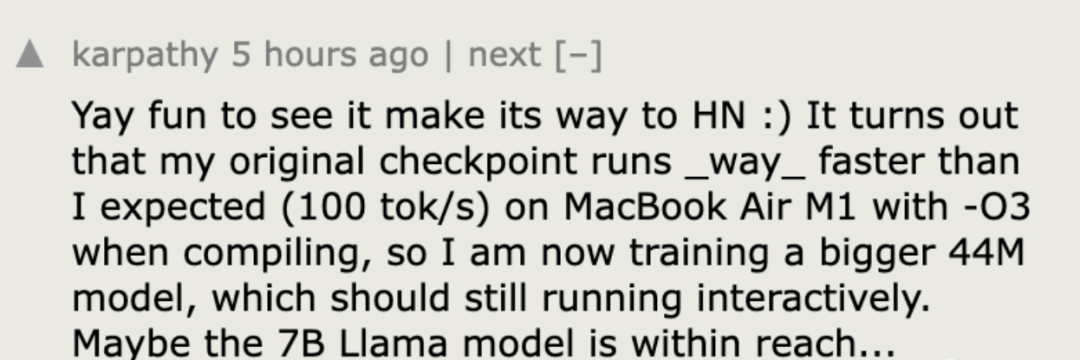GitHub：https://github.com/karpathy/llama2.c

## 6. 对标Llama 2，OpenAI开源模型G3PO已在路上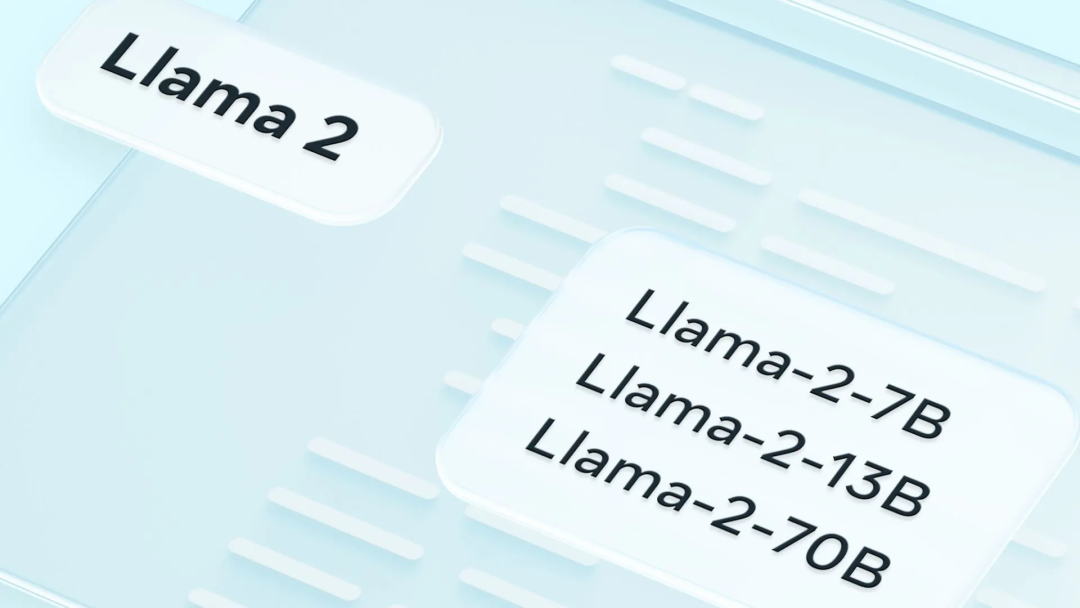GPT-4 虽然被一致认为能力领先，但 OpenAI 却并不是唯一一个引领方向的公司。在大模型领域，开源预训练模型一直被认为是重要的方向。

GPT-4 因为不提供开源版本而时常受到人们诟病，但 OpenAI 并不一定会坚持封闭的道路，其正在关注开源的影响。两个月前，有消息称该公司打算发布自己的开源模型以应对竞争。近日，据外媒 Information 报道，OpenAI 内部已有具体计划，未来即将开源的大模型代号为「G3PO」。• OpenAI 的团队规模较小，并不专注于推出为客户提供定制化大模型的商店。虽然这将是吸引开发者，并抵御 Meta 和 Google 的另一种途径。

• OpenAI 还希望打造个性化的 ChatGPT Copilot 助手。据消息人士透露，推出真正的「Copilot」将使 OpenAI 与微软直接竞争，有一些左右互搏的意思，而且这一努力「可能需要数年时间」。

## 7. 两万字长文详解：如何用C++从零实现神经网络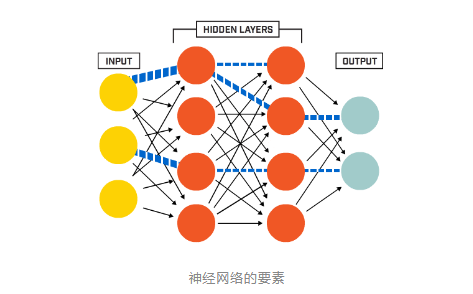• 神经元节点
• 层（layer）
• 权值（weights）
• 偏置项（bias）

Net类的设计
Net类——基于Mat



#ifndef NET\_H  #define NET\_H  #endif // NET\_H  #pragma once  #include \<iostream>  #include\<opencv2\\core\\core.hpp>  #include\<opencv2\\highgui\\highgui.hpp>  //#include\<iomanip>  #include"Function.h"  namespace liu  \{      class Net      \{      public:          std::vector\<int\> layer\_neuron\_num;          std::vector\<cv::Mat> layer;          std::vector\<cv::Mat> weights;          std::vector\<cv::Mat> bias;      public:          Net \{\};          \~Net \{\};          //Initialize net:genetate weights matrices、layer matrices and bias matrices          // bias default all zero          void initNet$std::vector\<int\> layer\_neuron\_num\_$;          //Initialise the weights matrices.          void initWeights$int type = 0, double a = 0., double b = 0.1$;          //Initialise the bias matrices.          void initBias$cv::Scalar\& bias$;          //Forward          void forward;          //Forward          void backward;      protected:          //initialise the weight matrix.if type =0,Gaussian.else uniform.          void initWeight$cv::Mat \&dst, int type, double a, double b$;          //Activation function          cv::Mat activationFunction$cv::Mat \&x, std::string func\_type$;          //Compute delta error          void deltaError;          //Update weights          void updateWeights;      \};  \}



• 每一层神经元数目（layerneuronnum）
• 层（layer）
• 权值矩阵（weights）
• 偏置项（bias）

• initNet()：用来初始化神经网络
• initWeights()：初始化权值矩阵，调用initWeight()函数
• initBias()：初始化偏置项
• forward()：执行前向运算，包括线性运算和非线性激活，同时计算误差
• backward()：执行反向传播，调用updateWeights()函数更新权值。

initNet()函数



//Initialize net    void Net::initNet(std::vector<int> layer_neuron_num_)    {        layer_neuron_num = layer_neuron_num_;        //Generate every layer.        layer.resize(layer_neuron_num.size());        for (int i = 0; i < layer.size(); i++)        {            layer[i].create(layer_neuron_num[i], 1, CV_32FC1);        }        std::cout << "Generate layers, successfully!" << std::endl;        //Generate every weights matrix and bias        weights.resize(layer.size() - 1);        bias.resize(layer.size() - 1);        for (int i = 0; i < (layer.size() - 1); ++i)        {            weights[i].create(layer[i + 1].rows, layer[i].rows, CV_32FC1);            //bias[i].create(layer[i + 1].rows, 1, CV_32FC1);            bias[i] = cv::Mat::zeros(layer[i + 1].rows, 1, CV_32FC1);        }        std::cout << "Generate weights matrices and bias, successfully!" << std::endl;        std::cout << "Initialise Net, done!" << std::endl;    }



initWeight()函数



//initialise the weights matrix.if type =0,Gaussian.else uniform.    void Net::initWeight(cv::Mat &dst, int type, double a, double b)    {        if (type == 0)        {            randn(dst, a, b);        }        else        {            randu(dst, a, b);        }    }    //initialise the weights matrix.    void Net::initWeights(int type, double a, double b)    {        //Initialise weights cv::Matrices and bias        for (int i = 0; i < weights.size(); ++i)        {            initWeight(weights[i], 0, 0., 0.1);        }    }





//Initialise the bias matrices.    void Net::initBias(cv::Scalar& bias_)    {        for (int i = 0; i < bias.size(); i++)        {            bias[i] = bias_;        }    }





#include"../include/Net.h"//<opencv2\opencv.hpp>using namespace std;using namespace cv;using namespace liu;int main(int argc, char *argv[]){    //Set neuron number of every layer    vector<int> layer_neuron_num = { 784,100,10 };    // Initialise Net and weights    Net net;    net.initNet(layer_neuron_num);    net.initWeights(0, 0., 0.01);    net.initBias(Scalar(0.05));    getchar();    return 0;}





weights[i].create(layer[i + 1].rows, layer[i].rows, CV_32FC1);





//Forward    void Net::forward()    {        for (int i = 0; i < layer_neuron_num.size() - 1; ++i)        {            cv::Mat product = weights[i] * layer[i] + bias[i];            layer[i + 1] = activationFunction(product, activation_function);        }    }


for循环里面的两句就分别是上面说的线型运算和激活函数的非线性运算。



//Activation function      cv::Mat Net::activationFunction$cv::Mat \&x, std::string func\_type$      \{          activation\_function = func\_type;          cv::Mat fx;          if $func\_type == "sigmoid"$          \{              fx = sigmoid$x$;          \}          if $func\_type == "tanh"$          \{              fx = tanh$x$;          \}          if $func\_type == "ReLU"$          \{              fx = ReLU$x$;          \}          return fx;      \}





//Backward    void Net::backward()    {        calcLoss(layer[layer.size() - 1], target, output_error, loss);        deltaError();        updateWeights();    }



• 第一个函数 calcLoss() 计算输出误差和目标函数，所有输出误差平方和的均值作为需要最小化的目标函数。
• 第二个函数 deltaError() 计算delta误差，也就是下图中delta1*df()那部分。
• 第三个函数 updateWeights() 更新权值，也就是用下图中的公式更新权值。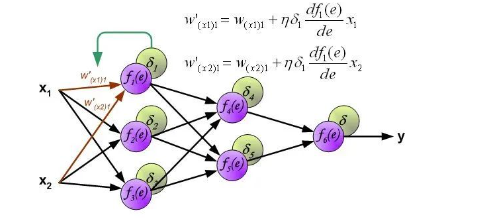//Update weights    void Net::updateWeights()    {        for (int i = 0; i < weights.size(); ++i)        {            cv::Mat delta_weights = learning_rate * (delta_err[i] * layer[i].t());            weights[i] = weights[i] + delta_weights;        }    }👇点击阅读原文进入官网0 评论
0 收藏
0